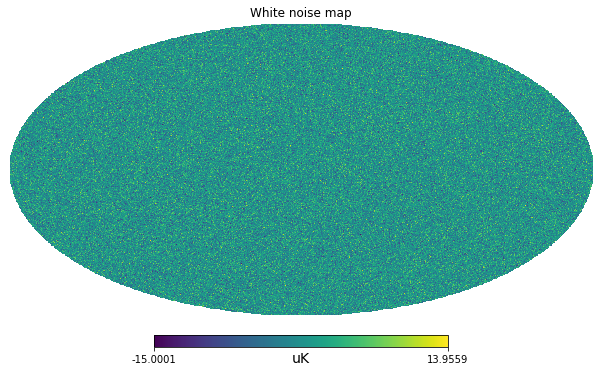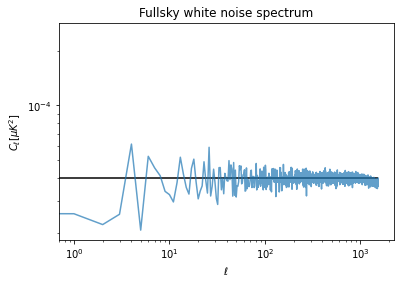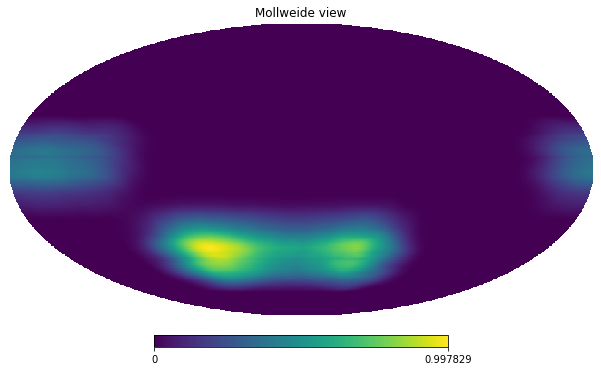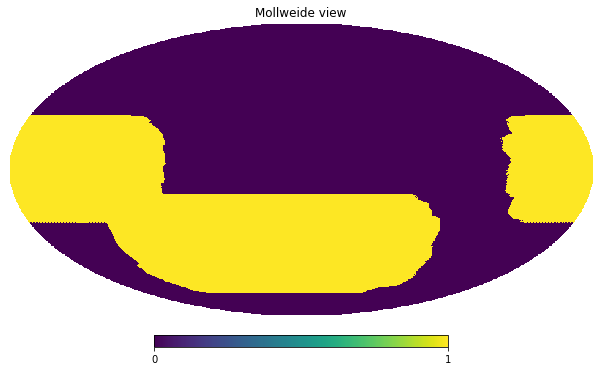import healpy as hp
import numpy as np
%matplotlib inline
import matplotlib.pyplot as plt
import astropy.units as u


In this series of notebooks, we will understand how to handle white noise in the case of an experiment with sky observations which are both not-uniform and have partial sky coverage.

Let's first start assuming a sensitivity of an experiment array of detectors of $10 \mu K \sqrt(s)$.

# Number based on Simons Observatory SAT UHF1 array of detectors

net = 10. * u.Unit("uK * sqrt(s)")


5 years with a efficiency of 20%:

integration_time_total = 5 * u.year * .2


## Uniform full sky survey

As a reference, let's first start with the trivial case of uniform full sky coverage, i.e. we spend the same amount of observation time in each pixel.

nside = 512
npix = hp.nside2npix(nside)

standard_deviation_per_pixel = (net / np.sqrt(integration_time_total/npix)).decompose()

standard_deviation_per_pixel

$3.1572473 \times 10^{-6} \; \mathrm{K}$
m = np.random.normal(scale = standard_deviation_per_pixel.value, size=npix) * standard_deviation_per_pixel.unit

m = m.to(u.uK)

hp.mollview(m, unit=m.unit, title="White noise map")## Power spectrum

Finally we can compute the angular power spectrum with anafast, i.e. the power as a function of the angular scales, from low $\ell$ values for large angular scales, to high $\ell$ values for small angular scales.

At low $\ell$ there is not much statistics and the power spectrum is biased, but if we exclude lower ells, we can have an estimate of the white noise $C_\ell$ coefficients. We can then compare with the theoretical power computed as:

$$C_\ell = \Omega_{pix}\sigma^2$$

Where: $\Omega_{pix}$ is the pixel are in square-radians and $\sigma$ is the white noise standard variance.

cl = hp.anafast(m)

cl[100:].mean()

3.9337952470928686e-05
m.std()

$3.1571259 \; \mathrm{\mu K}$
pixel_area = hp.nside2pixarea(nside)

white_noise_cl = (standard_deviation_per_pixel**2 * pixel_area).to(u.uK**2)

white_noise_cl

$3.9820426 \times 10^{-5} \; \mathrm{\mu K^{2}}$
plt.figure(figsize=(6,4))
plt.loglog(cl, label="Map power spectrum", alpha=.7)
plt.hlines(white_noise_cl.value, 0, len(cl), label="White noise level")
plt.title("Fullsky white noise spectrum")
plt.xlabel("$\ell$")
plt.ylabel("$C_\ell [\mu K ^ 2]$");## Survey

In case we are removing some pixels from a map, for example to mask out a strong signal (e.g. the Milky Way), our estimate of the power spectrum on the partial sky is lower. However we assume that the properties of the noise will be the same also in the masked region. At first order, for simple masks, we can just correct for the amplitude by dividing the power spectrum by the sky fraction.

hitmap_url = "https://portal.nersc.gov/project/sobs/so_mapsims_data/v0.2/healpix/ST0_UHF1_01_of_20.nominal_telescope_all_time_all_hmap.fits.gz"

!wget $hitmap_url  --2020-06-20 12:19:25-- https://portal.nersc.gov/project/sobs/so_mapsims_data/v0.2/healpix/ST0_UHF1_01_of_20.nominal_telescope_all_time_all_hmap.fits.gz Resolving portal.nersc.gov (portal.nersc.gov)... 128.55.201.128 Connecting to portal.nersc.gov (portal.nersc.gov)|128.55.201.128|:443... connected. HTTP request sent, awaiting response... 200 OK Length: 3212515 (3.1M) [application/x-gzip] Saving to: ‘ST0_UHF1_01_of_20.nominal_telescope_all_time_all_hmap.fits.gz.2’ ST0_UHF1_01_of_20.n 100%[===================>] 3.06M --.-KB/s in 0.1s 2020-06-20 12:19:25 (29.0 MB/s) - ‘ST0_UHF1_01_of_20.nominal_telescope_all_time_all_hmap.fits.gz.2’ saved [3212515/3212515]  hitmap = hp.read_map("ST0_UHF1_01_of_20.nominal_telescope_all_time_all_hmap.fits.gz")  /home/zonca/zonca/p/software/healpy/healpyvenv/lib/python3.7/site-packages/healpy/fitsfunc.py:352: UserWarning: If you are not specifying the input dtype and using the default np.float64 dtype of read_map(), please consider that it will change in a future version to None as to keep the same dtype of the input file: please explicitly set the dtype if it is important to you. "If you are not specifying the input dtype and using the default "  NSIDE = 512 ORDERING = NESTED in fits file INDXSCHM = IMPLICIT Ordering converted to RING  hitmap /= hitmap.max()  hp.mollview(hitmap)uniform_hitmap = hitmap > 0  hp.mollview(uniform_hitmap)sky_fraction = np.mean(uniform_hitmap)  sky_fraction  0.38526121775309247 assert sky_fraction == uniform_hitmap.sum() / len(uniform_hitmap)  integration_time_per_pixel_uniform_hitmap = (net / np.sqrt(integration_time_total/uniform_hitmap.sum())).decompose()  hp.ma(m_survey).compressed().std()  m_survey[hp.mask_bad(m_survey)] = 0  m_survey.std()  m_survey = np.ones_like(m) * hp.UNSEEN  m_survey[:npix//2] = np.random.normal(scale = standard_deviation_survey.value, size=npix//2) * standard_deviation.unit  hp.mollview(m_survey, unit=m.unit, title="White noise map")  cl_survey = hp.anafast(m_survey)  plt.figure(figsize=(6,4)) plt.loglog(cl, label="Map power spectrum", alpha=.7) plt.loglog(cl_survey*2, label="Map power spectrum (Masked)", alpha=.7) plt.hlines(white_noise_cl, 0, len(cl), label="White noise level") plt.xlabel("$\ell$") plt.ylabel("$C_\ell [\mu K ^ 2]$") plt.legend();  plt.figure(figsize=(6,4)) plt.loglog(cl, label="Map power spectrum", alpha=.7) plt.loglog(cl_masked, label="Map power spectrum (Masked)", alpha=.7) plt.hlines(white_noise_cl, 0, len(cl), label="White noise level") plt.xlabel("$\ell$") plt.ylabel("$C_\ell [\mu K ^ 2]$") plt.legend();  sky_fraction = hp.mask_good(m).sum() / len(m) print(sky_fraction)  plt.figure(figsize=(6,4)) plt.loglog(cl, label="Map power spectrum", alpha=.7) plt.loglog(cl_masked / sky_fraction, label="Map power spectrum (Masked) - corrected", alpha=.7) plt.hlines(white_noise_cl, 0, len(cl), label="White noise level") plt.xlabel("$\ell$") plt.ylabel("$C_\ell [\mu K ^ 2]\$")
plt.legend();